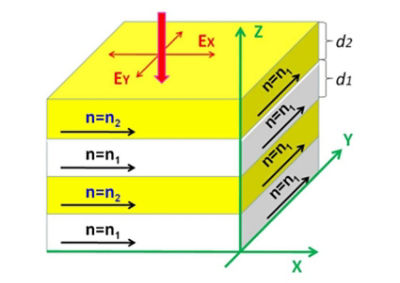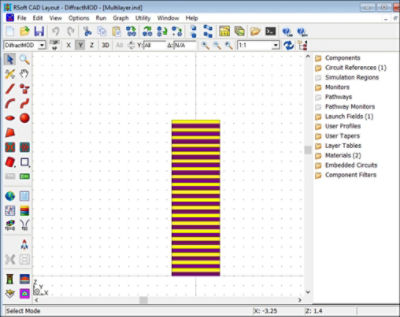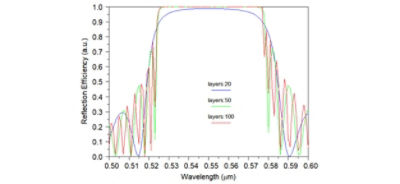# How to Design Reflective Polarizers for Display Backlight Recycling

## Tools Used: RSoft CAD Environment, DiffractMOD

Backlight recycling using a reflective polarizer improves the optical efficiency of flat displays. When the unpolarized backlight hits the reflective polarizer, one linear polarization (e.g. p-wave) will pass through while the other component (s-wave) will be reflected back for recycling. After hitting the rough surface of the diffuser, the s-wave is depolarized. Upon reflection from the diffusive reflector, the p-wave is transmitted, and s-wave is reflected again for recycling. In each cycle, about half of the incident light passes through the polarizer. After 3 to 5 cycles, about 50%–60% of the s-wave is converted to p-wave.

Several types of reflective polarizers have been developed. This includes a multilayer thin film reflective polarizer, also known as DBEF (dual brightness enhancement film) , , which provides a mechanism to recycle the backlight before it enters the display panel -- resulting in improved optical efficiency. The DBEF manufacturing technology is mature and commonly used in commercial products, especially for battery-powered mobile displays such as cell phones and notebook computers.Figure 1: The structure of a multilayer birefringent polymereiec reflective polarizer

Figure 1 shows the device structure of a multilayer birefringent reflective polarizer consisting of dozens of alternative layers (materials 1 and 2). Material 1 is an isotropic polymer and material 2 is a positive birefringence uniaxial polymer. There is a large refractive index difference between the two materials in the x axis. The refractive indices in the y and z axes are substantially matched. In this example, z represents the light propagating direction perpendicular to the layers.

Meanwhile, the optical thickness of each layer is controlled to be a quarter of a center wavelength. Therefore, Bragg reflection could be established for the polarization component along the x axis. For polarization along the y axis, the light sees a thick slab with uniform refractive index and is transmitted with high efficiency.

High-reflectance polarizers can be easily modeled and optimized with the RSoft DiffractMOD RCWA tool. In this application note, we will outline an example of how to do this.

In the example model shown in Figure 2, NOA81 and BL038 are the two materials used for the multilayer polarizer. For convenience, we designated the isotropic material’s refractive index as N1 and the uniaxial material’s refractive index in x direction as N2. The refractive indices can be expressed with following equation:

N1,2 = A1,2 + B1,22 + C1,2 / λ4

Here A1=1.5519, B1=0.0036um2, C1=0.00049um4
A2=1.74775, B2=0.01184um2, C1=0.00303um4

are found via fitting for the two materials. Each layer has a quarter-wave optical thickness for the light polarized in the x direction.

As shown in Figure 2, the structure can be quickly set up in the RSoft CAD interface using the dynamic array feature:

• The first step is to set up a 1-period layer .ind file and define the two materials with above isotropic and anisotropic properties as a unit cell.
• The next step is to set up a multilayer array along the z direction using a dynamic array. The number of the layers can be changed by the periods in the z direction.Figure 2: Multilayer structure setups in RSoft CAD

Simulating the multilayer film with DiffractMOD is very simple and fast. Only the 0th harmonic is needed for the calculation. The reflection diffraction efficiency versus wavelength corresponding to 20, 50 and 100 periods are plotted in Figure 3. A strong reflection spectrum around 0.55um wavelength shows the Bragg reflection is established. The higher number of layers corresponds to higher reflection efficiency and wider bandwidth. For s-polarization, the multilayer won’t show Bragg reflection effects.Figure 3: Simulated Reflection Efficiencies of x-polarization (p-polarization)